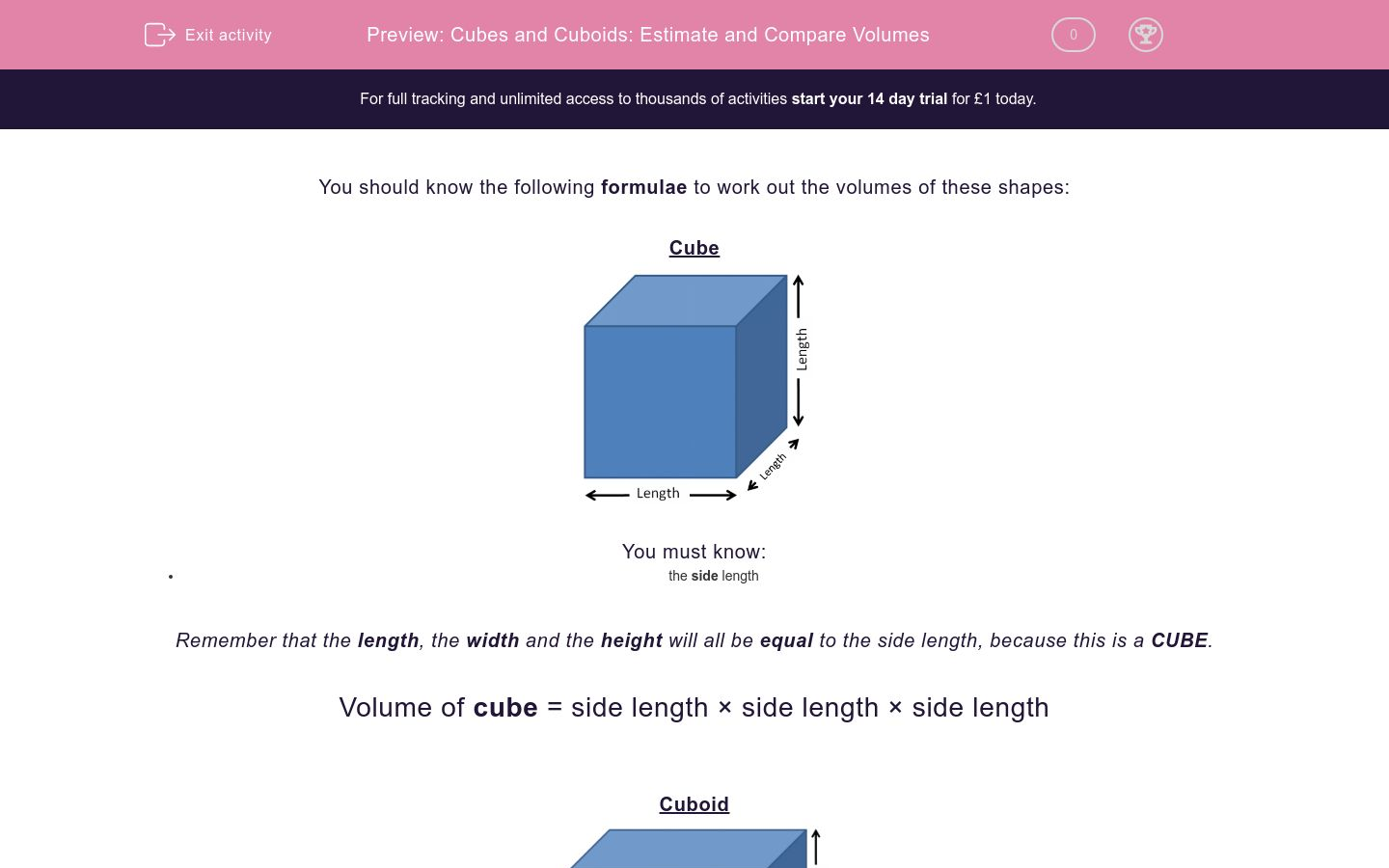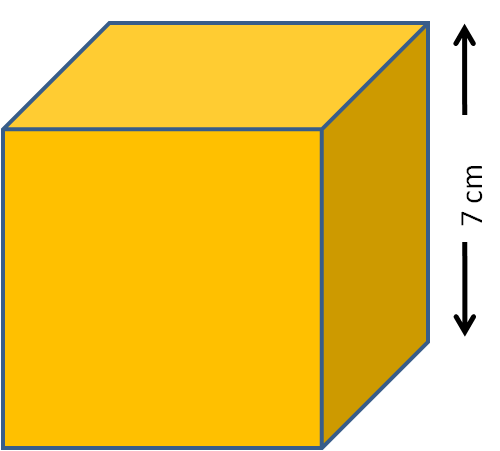# Cubes and Cuboids: Estimate and Compare Volumes

In this worksheet, students must estimate and compare the volumes of the given cubes and cuboids.Key stage:  KS 2

Curriculum topic:   Maths and Numerical Reasoning

Curriculum subtopic:   Volume and Capacity

Difficulty level:#### Worksheet Overview

You should know the following formulae to work out the volumes of these shapes:

CubeYou must know:

• the side length

Remember that the length, the width and the height will all be equal to the side length, because this is a CUBE.

Volume of cube = side length × side length × side length

CuboidYou must know:

• the length
• the width
• the height

Volume of cuboid = length × width × height

Example 1

How many 1 cm3 cubes will fit into this cuboid?The length is 15 cm, the width is 4 cm and the height is 8 cm.

Volume of cuboid  = length × width × height = 15 × 4 × 8 = 60 × 8 = 480 cm3.

So 480 cubes will fit.

Example 2

Calculate the volume of this cube in mm3.The side length is 7 cm, so the width and the height are also 7 cm.

This will be 70 mm.

NB: We want the answer in mm3 which is why we should work in mm not cm.

Volume of cube = side length × side length × side length = 70 × 70 × 70 = 49 × 7 × 10 × 10 × 10 = 343 × 1000 = 343000 mm3.

NB: The length, the width and the height are all interchangeable with each other, depending on how you look at a cuboid.

### What is EdPlace?

We're your National Curriculum aligned online education content provider helping each child succeed in English, maths and science from year 1 to GCSE. With an EdPlace account you’ll be able to track and measure progress, helping each child achieve their best. We build confidence and attainment by personalising each child’s learning at a level that suits them.

Get started